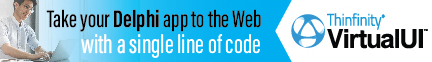DelphiBasicsRandomRangeFunction Generate a random integer number within a supplied range Math unit
 function RandomRange ( const RangeFrom, RangeTo : Integer ) : Integer;
Description
The RandomRange function generates a random Integer number within the range RangeFrom to RangeTo inclusively. *

This provides a more convenient version of the System unit Random function.

Both use a pseudo random number sequence of 232 values. Each time you run your program, the values generated will be the same, unless you reposition the generator to a different part of the sequence using the Randomize or RandSeed functions.
Related commands
 Random Generate a random floating point or integer number Randomize Reposition the Random number generator next value RandSeed Reposition the Random number generator next value
Author linksDownload this web site as a Windows program.

 Example code : Generate random numbers in a very small range var   i : Integer; begin  // Show 5 random numbers in the range 652 to 656   for i := 1 to 5 do     ShowMessage('Random number : '+IntToStr(RandomRange(652, 656))); end; Show full unit code Random number = 652   Random number = 652   Random number = 655   Random number = 652   Random number = 653
 Delphi Programming © Neil Moffatt 2002 - 2020. All rights reserved.  |  Home Page